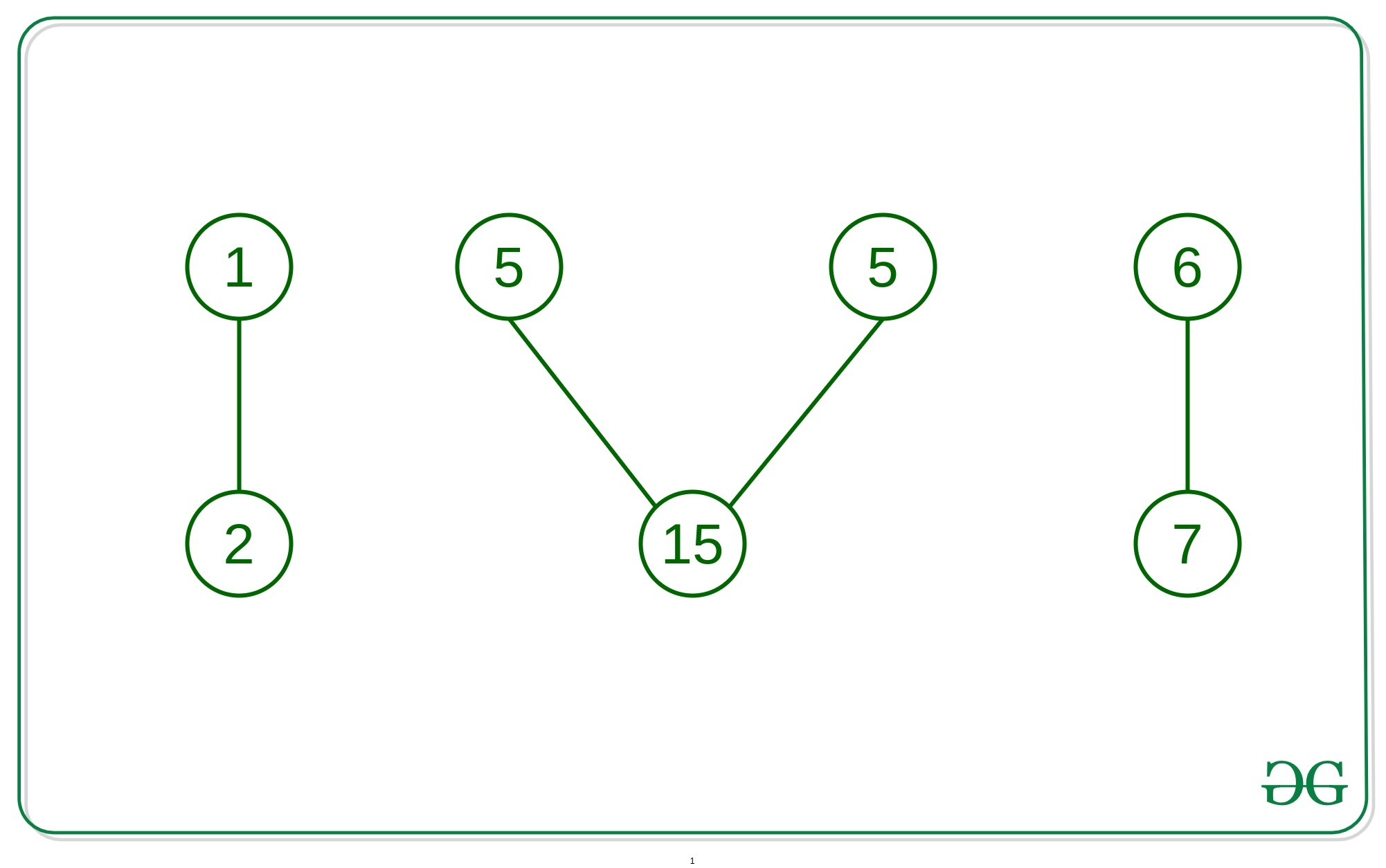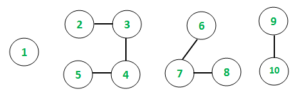# Check if longest connected component forms a palindrome in undirected graph

Given an undirected graph with V vertices and E edges, the task is to check that if the largest connected component of the graph forms a palindrome in the undirected graph.

Examples:

Input:Output:
Longest connected component is Palindrome
Explanation:
Longest connected component is {5, 15, 5}
which forms an palindrome by values.

Input:Output:
Longest connected component is not a Palindrome
Explanation:
Longest chain is {2, 3, 4, 5} which is not a palindrome.

## Recommended: Please try your approach on {IDE} first, before moving on to the solution.

Approach: The idea is to use Depth First Search Traversal to keep track of the connected components in the undirected graph. At each traversal, if the current length of the connected component is greater than the length of the global length of the connected component then update the longest connected component. Finally, check the longest connected component forms a palindrome.

Below is the implementation of the above approach:

## C++

 `// C++ implementation to check if ` `// longest connected component is  ` `// palindrome in undirected graph ` ` `  `#include ` ` `  `using` `namespace` `std; ` ` `  `// Function to traverse the undirected ` `// graph using the Depth first traversal ` `void` `depthFirst(``int` `v, vector<``int``> graph[], ` `                ``vector<``bool``>& visited, ` `                ``vector<``int``>& storeChain) ` `{ ` `    ``// Marking the visited  ` `    ``// vertex as true ` `    ``visited[v] = ``true``; ` ` `  `    ``// Store the connected chain ` `    ``storeChain.push_back(v); ` ` `  `    ``for` `(``auto` `i : graph[v]) { ` `        ``if` `(visited[i] == ``false``) { ` `             `  `            ``// Recursive call to  ` `            ``// the DFS algorithm ` `            ``depthFirst(i, graph,  ` `               ``visited, storeChain); ` `        ``} ` `    ``} ` `} ` ` `  `// Function to check that the connected ` `// component forms a palindrome ` `void` `checkPalin(``int` `arr[], ``int` `n) ` `{ ` `    ``// Container to store the frequency ` `    ``// of each occurring element ` `    ``map<``int``,``int``> frequency; ` `  `  `    ``// Container to visit elements ` `    ``unordered_set<``int``> element; ` `  `  `    ``for``(``int` `i = 0; i < n; i ++) ` `    ``{ ` `        ``// If element has not been visited already ` `        ``// the element is inserted with freq = 1 ` `        ``// frequency of occurrence, if visited ` `        ``// already, then frequency is updated ` `        ``if``(!(element.find(arr[i]) != element.end())) ` `        ``{ ` `            ``frequency.insert({arr[i], 1}); ` `        ``} ` `        ``else` `        ``{ ` `            ``frequency[arr[i]] ++; ` `        ``} ` `        ``element.insert(arr[i]); ` `    ``} ` `  `  `    ``// Variable to store final result ` `    ``int` `result = 1; ` `  `  `    ``// For even array size, all the elements ` `    ``// are checked for even occurrences, if ` `    ``// odd array size, then it is checked if ` `    ``// a single element with odd frequency ` `    ``// is present or not ` `    ``if``(n % 2 == 0) ` `    ``{ ` `        ``for``(``auto` `i: frequency) ` `        ``{ ` `            ``if``(i.second % 2 != 0) ` `            ``{ ` `                ``result = 0; ` `                ``break``; ` `            ``} ` `        ``} ` `    ``} ` `    ``else` `    ``{ ` `        ``int` `countFreq = 0; ` `        ``for``(``auto` `i: frequency) ` `        ``{ ` `            ``if``(i.second % 2 != 0) ` `            ``{ ` `                ``countFreq ++; ` `            ``} ` `        ``} ` `        ``if``(countFreq != 1) ` `            ``result = 0; ` `    ``} ` `  `  `    ``// Printing the final result ` `    ``if``(result) ` `        ``cout << ``"Longest connected component is Palindrome"``; ` `    ``else` `        ``cout << ``"Longest connected component not a Palindrome"``; ` `} ` ` `  `// Function to check that longest connected ` `// component forms a palindrome ` `void` `longestConnectionPalin( ` `    ``vector<``int``> graph[], ``int` `vertices, ` `                   ``vector<``int``> values) ` `{ ` `    ``// Initializing boolean array ` `    ``// to mark visited vertices ` `    ``vector<``bool``> visited(10001, ``false``); ` ` `  `    ``// maxChain stores the  ` `    ``// maximum chain size ` `    ``int` `maxChain = 0; ` ` `  `    ``// Container to store longest chain ` `    ``vector<``int``> maxStoreChain; ` ` `  `    ``// Following loop invokes DFS algorithm ` `    ``for` `(``int` `i = 1; i <= vertices; i++) { ` `        ``if` `(visited[i] == ``false``) { ` `             `  `            ``// Variable to hold  ` `            ``// temporary length ` `            ``int` `sizeChain; ` ` `  `            ``// Container to store each chain ` `            ``vector<``int``> storeChain; ` ` `  `            ``// DFS algorithm ` `            ``depthFirst(i, graph, visited, storeChain); ` ` `  `            ``// Variable to hold each chain size ` `            ``sizeChain = storeChain.size(); ` ` `  `            ``if` `(sizeChain > maxChain) { ` `                ``maxChain = sizeChain; ` `                ``maxStoreChain = storeChain; ` `            ``} ` `        ``} ` `    ``} ` ` `  `    ``// Container to store values ` `    ``// of vertices of longest chain ` `    ``int` `longChainValues[maxChain+1]; ` `  `  `    ``// Storing the values of longest chain ` `    ``for``(``int` `i = 0; i < maxChain; i ++) ` `    ``{ ` `        ``int` `temp = values[maxStoreChain[i]-1]; ` `        ``longChainValues[i] = temp; ` `    ``} ` `  `  `    ``// Function call to check for Palindrome ` `    ``checkPalin(longChainValues, maxChain); ` `} ` ` `  `// Driver function to test above function ` `int` `main() ` `{ ` `    ``// Initializing graph in the form of adjacency list ` `    ``vector<``int``> graph; ` ` `  `    ``// Defining the number of edges and vertices ` `    ``int` `E, V; ` `    ``E = 4; ` `    ``V = 7; ` ` `  `    ``// Assigning the values for each ` `    ``// vertex of the undirected graph ` `    ``vector<``int``> values; ` `    ``values.push_back(10); ` `    ``values.push_back(25); ` `    ``values.push_back(5); ` `    ``values.push_back(15); ` `    ``values.push_back(5); ` `    ``values.push_back(20); ` `    ``values.push_back(0); ` ` `  `    ``// Constructing the undirected graph ` `    ``graph.push_back(2); ` `    ``graph.push_back(1); ` `    ``graph.push_back(4); ` `    ``graph.push_back(3); ` `    ``graph.push_back(5); ` `    ``graph.push_back(3); ` `    ``graph.push_back(7); ` `    ``graph.push_back(6); ` ` `  `    ``longestConnectionPalin(graph, V, values); ` `    ``return` `0; ` `} `

Output:

```Longest connected component is Palindrome
```

Time Complexity: O(V + E)My Personal Notes arrow_drop_upCheck out this Author's contributed articles.

If you like GeeksforGeeks and would like to contribute, you can also write an article using contribute.geeksforgeeks.org or mail your article to contribute@geeksforgeeks.org. See your article appearing on the GeeksforGeeks main page and help other Geeks.

Please Improve this article if you find anything incorrect by clicking on the "Improve Article" button below.